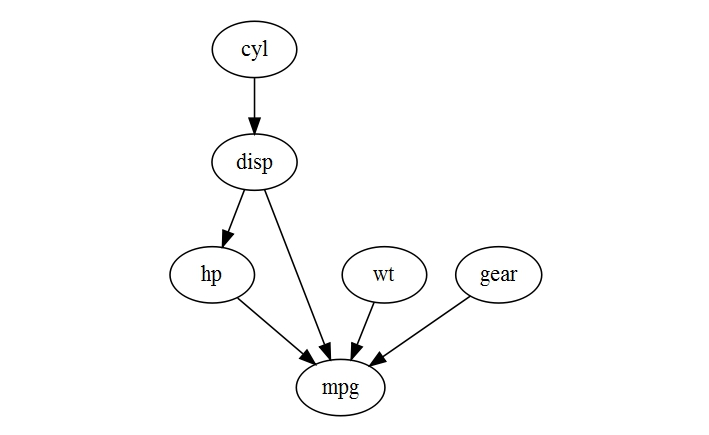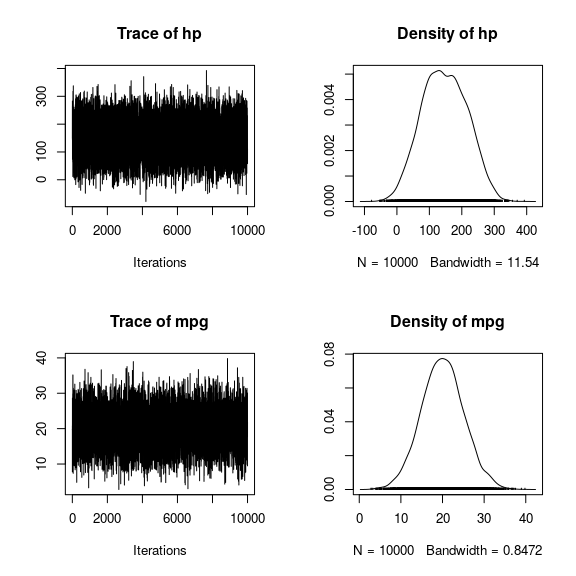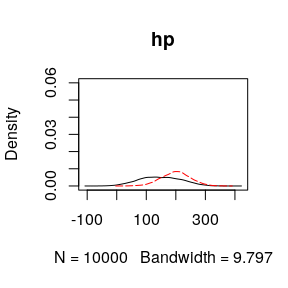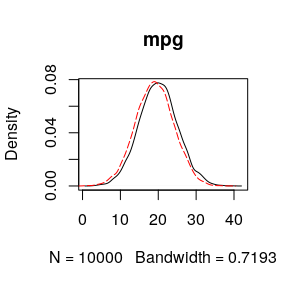# Getting Started with HydeNet

#### 2020-05-15

HydeNet is a package intended to facilitate modeling of hybrid Bayesian networks and influence diagrams (a.k.a. decision networks). A Bayesian network, formally defined, is a joint probability distribution for a set of random variables for which the set of conditional independencies can be represented using a directed acyclic graph. Each node represents a random variable, and each link represents the direction of influence between two nodes. The probability distribution for any given node is defined as conditional (only) on its parent nodes, and every node is conditionally independent from all of its non-descendants given data on its parent nodes.

Bayesian networks are powerful dynamic inference models that allow for updating predictions of unobserved nodes in the network as evidence is collected. They can be constructed using expert knowledge, or by using structure learning algorithms. HydeNet assumes the first approach: all networks are manually constructed through collaboration with subject matter experts. Users interested in structure learning problems — which are popular in -omics applications, for example — are encouraged to try the bnlearn package by Marco Scutari.

Influence diagrams (or decision networks) are extended Bayesian networks which incorporate two additional types of nodes: decision nodes and utility nodes. Decision nodes are assumed to be manipulable by the actor, while utility nodes are deterministic functions of one or more parent nodes which produce numeric outputs. When there are multiple decision nodes in the network, specific combinations of decision node values are referred to as policies. We can use these networks to study the distribution of utility as it relates to various decision-making policies, and to conduct value-of-information analyses. An overview of using HydeNet for decision network analysis is provided in the “Decision Networks” vignette.

Packages exist for exact inference on standard Bayesian networks (e.g., gRain, gRim) when the networks do not have decision/utility nodes and fit any of the following criteria:

• All nodes are discrete/categorical in nature
• All nodes are Gaussian in nature
• All nodes are either Gaussian or discrete/categorical in nature (i.e., conditional linear gaussian models)

Exact inference on Bayesian networks is an NP-hard problem. When networks become large, either in the number of parameters in the number of nodes, exact inference algorithms such as junction tree are computationally expensive. In these situations, approximate inference may be an appealing alternative. Several methods for approximate inference on Bayesian networks exist. Popular methods include Markov Chain Monte Carlo sampling, application of the EM algorithm, and logic sampling (which is implemented in bnlearn). HydeNet implements Markov Chain Monte Carlo inference through an interface to JAGS.

Fundamentally, HydeNet works by writing a JAGS script according to the structure and parameterization of your graphical model, and calling JAGS with that script.

## HydeNet Installation

If you don’t already have JAGS installed, you will receive an error when HydeNet tries to load the rjags package. rjags searches your system for an installation of JAGS and throws an error if none is found. You will need to download and install JAGS before using HydeNet.

Installing HydeNet from CRAN is straightforward and can be done with the usual syntax:

install.packages("HydeNet")

If you wish to install a more recent or development version from GitHub, you may need to point R to the BioConductor repositories.

setRepositories(ind=1:2)
devtools::install_github("nutterb/HydeNet")

## A Simple Bayesian Network Analysis

First we load the library, and do some preliminary manipulation of the mtcars data frame:

library(HydeNet)

mtcars2 <- transform(mtcars,
cyl = factor(cyl),
gear=factor(gear),
am = factor(am))

### Network Construction

The first step in the analysis is to set up the network structure. This is done using the HydeNetwork() function. The formula method for HydeNetwork() uses syntax similar to the dag() command in the gRbase library:

carNet <- HydeNetwork(~ cyl
+ disp | cyl
+ hp | disp
+ wt
+ gear
+ mpg | disp*hp*wt*gear,
data=mtcars2)

In the above, we have passed the training data frame mtcars2 to be used for populating the parameters of the network. But, there are several ways to populate the node distributions:

1. automatically, by calling HydeNetwork() with a training dataset (as in the above);
2. manually, by calling HydeNetwork() with a list of model objects; and
3. manually, by specifying only the network structure in the call to HydeNetwork() and then subsequently using the functions setNode() and setNodeModels() to specify distributions for each individual node.

In the automatic approach, estimation of the conditional probability distribution for each node (given its parent nodes) is performed using different models, depending on the nature of the node and the nature of its parent nodes. For example, conditional distributions of categorical nodes with all categorical parents are estimated using tabulation (see help('cpt')), while conditional distributions for categorical nodes with at least one continuous parent node are estimated using glm(, family="binomial").

There are many options for manual specification of node distributions. Please refer to our “Working with HydeNet Objects” vignette (vignette("WorkingWithHydeNetObjects", package = "HydeNet"))for all the details on how to use HydeNetwork(), setNode() and setNodeModels() to create and populate network objects.

### Network Visualization

We have implemented a plot method for HydeNetwork objects, using the DiagrammeR library by Richard Iannone et al. While not implemented in this simple example, there are different default node shapes and colors for random variable nodes, deterministic nodes, decision nodes and utility nodes. See our “Building and Customizing HydeNet Plots” vignette (vignette("HydeNetPlots", package = "HydeNet"))for details on customization.

plot(carNet)## Interfacing with JAGS

HydeNet interfaces with JAGS through the rjags package. Given a fully-specified HydeNetwork model, the function writeNetworkModel() will create the JAGS model script (by calling the writeJagsModel() function for each node, which creates each node’s portion of the script). Here’s an example (note, as indicated by the need to use a triple colon, that writeJagsModel() is not an exported function to the HydeNet namespace):

HydeNet:::writeJagsModel(carNet, node = "cyl")
##  "pi.cyl <- 0.34375; pi.cyl <- 0.21875; pi.cyl <- 0.4375 \ncyl ~ dcat(pi.cyl)"
HydeNet:::writeJagsModel(carNet, node = "mpg")
##  "mpg ~ dnorm(35.02449 + 0.00858 * disp + 1.88654 * (gear == 2) + 2.38977 * (gear == 3) + -0.04032 * hp + -3.75433 * wt, 0.14288)"
writeNetworkModel(carNet, pretty = TRUE)
## model{
##    pi.cyl <- 0.34375; pi.cyl <- 0.21875; pi.cyl <- 0.4375
## cyl ~ dcat(pi.cyl)
##    disp ~ dnorm(105.13636 + 78.17792 * (cyl == 2) + 247.96364 * (cyl == 3), 0.00038)
##    hp ~ dnorm(45.73453 + 0.43755 * disp, 0.00055)
##    wt ~ dnorm(3.21725, 1.04452)
##    pi.gear <- 0.46875; pi.gear <- 0.375; pi.gear <- 0.15625
## gear ~ dcat(pi.gear)
##    mpg ~ dnorm(35.02449 + 0.00858 * disp + 1.88654 * (gear == 2) + 2.38977 * (gear == 3) + -0.04032 * hp + -3.75433 * wt, 0.14288)
## }

In practice, though, the function compileJagsModel() (or compileDecisionModel(), if the network contains decision and utility nodes) will typically be called. This function is a wrapper for the jags.model() function in the rjags library which, in addition to the JAGS model script, passes any conditional probability tables from the network model into JAGS as arrays. (Note: all other arguments to jags.model(), such as the number of chains initial values, and the number of iterations for adaptation are passed through to the function.)

The functions compileJagsModel() and compileDecisionModel() essentially return augmented objects of class jags. We label these objects with the class compiledHydeNetwork.

carNet1 <- compileJagsModel(carNet)
## Compiling model graph
##    Resolving undeclared variables
##    Allocating nodes
## Graph information:
##    Observed stochastic nodes: 0
##    Unobserved stochastic nodes: 6
##    Total graph size: 49
##
## Initializing model

Evidence on individual nodes can also be entered with compileJagsModel(), thus facilitating dynamic inference as data are observed. See the description of the data argument in help(rjags::jags.model) for details.

carNet2 <- compileJagsModel(carNet, data = list(cyl = "8") )
## Compiling model graph
##    Resolving undeclared variables
##    Allocating nodes
## Graph information:
##    Observed stochastic nodes: 1
##    Unobserved stochastic nodes: 5
##    Total graph size: 49
##
## Initializing model

As a matter of convenience, HydeNet can also translate labels into the numeric codes

carNet3 <- compileJagsModel(carNet, data=list(cyl="8"))
## Compiling model graph
##    Resolving undeclared variables
##    Allocating nodes
## Graph information:
##    Observed stochastic nodes: 1
##    Unobserved stochastic nodes: 5
##    Total graph size: 49
##
## Initializing model

## MCMC Inference

MCMC sampling is performed by calling HydePosterior(). This function calls coda.samples() from the rjags library and returns an augmented mcmc.list object. MCMC diagnostics and inference from this point onward are performed in the same manner as would typically be done when using rjags.

post1 <- HydePosterior(carNet1,
variable.names = c("cyl","hp","mpg"),
n.iter = 10000,
bind=FALSE)
## HydePoseterior has been deprecated and replaced by HydeSim
post2 <- HydePosterior(carNet2,
variable.names = c("cyl","hp","mpg"),
n.iter = 10000,
bind = FALSE)
## HydePoseterior has been deprecated and replaced by HydeSim
str(post1, max.level = 3)
## List of 4
##  $codas :List of 1 ## ..$ : 'mcmc' num [1:10000, 1:3] 3 3 3 2 3 3 3 1 1 1 ...
##   .. ..- attr(*, "dimnames")=List of 2
##   .. ..- attr(*, "mcpar")= num [1:3] 1 10000 1
##   ..- attr(*, "class")= chr "mcmc.list"
##  $observed : NULL ##$ dag      : num [1:6, 1:6] 0 0 0 0 0 0 1 0 0 0 ...
##   ..- attr(*, "dimnames")=List of 2
##   .. ..$: chr [1:6] "cyl" "disp" "hp" "wt" ... ## .. ..$ : chr [1:6] "cyl" "disp" "hp" "wt" ...
##  $factorRef:List of 3 ## ..$ cyl :'data.frame': 3 obs. of  2 variables:
##   .. ..$value: int [1:3] 1 2 3 ## .. ..$ label: chr [1:3] "4" "6" "8"
##   ..$gear:'data.frame': 3 obs. of 2 variables: ## .. ..$ value: int [1:3] 1 2 3
##   .. ..$label: chr [1:3] "3" "4" "5" ## ..$ am  :'data.frame': 2 obs. of  2 variables:
##   .. ..$value: int [1:2] 1 2 ## .. ..$ label: chr [1:2] "0" "1"
##  - attr(*, "class")= chr "HydeSim"
plot(post1$codas[,c("hp","mpg")])We have included a convenience function called bindPosterior() than can be used to combine multiple chains’ samples into a single data frame with all factors re-mapped to their original non-numeric values. Below, we apply the function to obtain posterior densities under each of the two scenarios (no observation of other nodes and after observation of cyl = “8”, respectively): bp1 <- bindPosterior(post1) ## bindPosterior is deprecated and replaced by bindSim bp2 <- bindPosterior(post2) ## bindPosterior is deprecated and replaced by bindSim head(bp1) ## cyl hp mpg chain_index obs_index ## 1 8 148.6793 20.793012 1 1 ## 2 8 194.3766 20.793579 1 1 ## 3 8 265.2868 7.782705 1 1 ## 4 6 163.6596 19.169470 1 1 ## 5 8 282.8805 13.937855 1 1 ## 6 8 258.2580 28.651105 1 1 head(bp2) #notice cyl = "8" for all samples ## cyl hp mpg chain_index obs_index ## 1 8 173.0702 22.68113 1 1 ## 2 8 191.8951 23.05469 1 1 ## 3 8 215.1883 19.96147 1 1 ## 4 8 175.9967 29.81924 1 1 ## 5 8 171.5972 23.19321 1 1 ## 6 8 125.0177 22.06108 1 1 plot(density(bp1$hp), ylim=c(0,0.06), main = "hp");
lines(density(bp2$hp), col="red", lty=5)plot(density(bp1$mpg), main = "mpg");
lines(density(bp2\$mpg), col="red", lty=5)## Global HydeNet Options

There are a couple of global options HydeNet establishes that you should be aware of.

### Hyde_fitModel

The Hyde_fitModel controls the behavior of setNode function. If FALSE, the model described by the arguments will not be fit until the JAGS model statement is written by writeNetworkModel.

The option can be set by using the code below. The default setting is FALSE.

options(Hyde_fitModel=FALSE)

Realistically, the time spent to fit the model will be spent one way or another, and it’s a matter of preference for where you spend that time. If you wish to do it all at once when calling writeNetworkModel, set the option to FALSE. Your printed output when reviewing a network will appear as follows:

## A Probabilistic Graphical Network
## Has data attached: Yes
##
## treat | d.dimer * angio
## dbern(prob = fromData)
## glm: treat ~ poly(d.dimer, 2) + angio

Notice that the mu and tau parameters are not explicitly defined, but only give a notice that they will be estimated from the data.

If the Hyde_fitModel option is set to TRUE, the output would appear as follows:

## A Probabilistic Graphical Network
## Has data attached: Yes
##
## treat | d.dimer * angio
## dbern(prob = fromData, p = treat ~ ilogit(-1.40357 + 1.74183*angio + 81.96278 + -3.60779))
## glm: treat ~ poly(d.dimer, 2) + angio

### Hyde_maxDigits

This options, set to 5 by default, controls the number of decimal places printed in the JAGS model code. It can be increased or decreased depending on the level of precision you need or want in your estimates.

library(HydeNet)
data(PE, package='HydeNet')
autoNet <- HydeNetwork(~ wells
+ pe | wells
+ d.dimer | pregnant*pe
+ angio | pe
+ treat | d.dimer*angio
+ death | pe*treat,
data = PE)
writeNetworkModel(autoNet, pretty=TRUE)
## model{
##    wells ~ dnorm(3.7942, 0.39754)
##    pe ~ dbern(ilogit(-3.90355 + 0.5757 * wells))
##    d.dimer ~ dnorm(210.24251 + 68.37938 * (pe == 1) + 29.29496 * (pregnant == 2), 0.00112)
##    pi.pregnant <- 0.9014; pi.pregnant <- 0.0986
## pregnant ~ dcat(pi.pregnant)
##    pi.angio <- cpt.angio[(pe+1), ]
##    angio ~ dcat(pi.angio)
##    treat ~ dbern(ilogit(-5.89316 + 1.73354 * angio + 0.01994 * d.dimer))
##    pi.death <- cpt.death[(pe+1), (treat+1), ]
##    death ~ dcat(pi.death)
## }

If 5 digits is too much for your taste:

library(HydeNet)
options(Hyde_maxDigits=2)
data(PE, package='HydeNet')
autoNet <- HydeNetwork(~ wells
+ pe | wells
+ d.dimer | pregnant*pe
+ angio | pe
+ treat | d.dimer*angio
+ death | pe*treat,
data = PE)
writeNetworkModel(autoNet, pretty=TRUE)
## model{
##    wells ~ dnorm(3.79, 0.4)
##    pe ~ dbern(ilogit(-3.9 + 0.58 * wells))
##    d.dimer ~ dnorm(210.24 + 68.38 * (pe == 1) + 29.29 * (pregnant == 2), 0)
##    pi.pregnant <- 0.9; pi.pregnant <- 0.1
## pregnant ~ dcat(pi.pregnant)
##    pi.angio <- cpt.angio[(pe+1), ]
##    angio ~ dcat(pi.angio)
##    treat ~ dbern(ilogit(-5.89 + 1.73 * angio + 0.02 * d.dimer))
##    pi.death <- cpt.death[(pe+1), (treat+1), ]
##    death ~ dcat(pi.death)
## }

This note applies to R versions less than 3.2.

You may notice throughout the vignettes that we tend to call the print function explicitly. That is, we use print(object) instead of simply submitting object to the console. The HydeNet objects can get pretty large, and the implicit printing can become pretty slow as a result. Consider the following comparison:

> #* Object based on a list of models
> g1 <- lm(wells ~ 1, data=PE)
> g2 <- glm(pe ~ wells, data=PE, family="binomial")
> g3 <- lm(d.dimer ~ pe + pregnant, data=PE)
> g4 <- xtabs(~ pregnant, data=PE)
> g5 <- glm(angio ~ pe, data=PE, family="binomial")
> g6 <- glm(treat ~ d.dimer + angio, data=PE, family="binomial")
> g7 <- glm(death ~ pe + treat, data=PE, family="binomial")

> bagOfModels <- list(g1,g2,g3,g4,g5,g6,g7)
> bagNet <- HydeNetwork(bagOfModels)

> #* Time to print bagNet implicitly
> a <- Sys.time()
> bagNet
> b <- Sys.time()
> b-a
Time difference of 33.53736 secs

> #* Time to print bagNet explicitly
> a <- Sys.time()
> print(bagNet)
> b <- Sys.time()
> b-a
Time difference of 0 secs